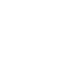Inspired by the likes of Tokyo Flash watches, I started thinking about some concept watch designs that would force the wearer to work the old grey matter in order to work out what time it was.

Some offer more precision that others, and some are easier to read, but all are definitely not your average analog or digital watch.

### 1. Fraction Clock

Granted, this is a simple digital watch so it’s not hard to see what time it is.

However, what this watch will do is to chime once in each one hour period — not necessarily on the hour but based on the associated fraction for that hour.The minutes and seconds are calculated from the rounded decimal equivalents of each fraction.

So, where hour one is 1/1 or simply 1am/1pm, the seventh hour is 1/7 or 07:14:29.

Interesting that the sixth hour becomes 06:17:07 because there would be no such time as 06:16:67.

### 2. Rainbow watch

The inner circle, divided into four sections, denotes the hour.

Colours red through indigo (no violet) denote hours 1-6, 7-12, 13-18 or (without indigo) 19-23, with the colour appearing in the appropriate section – top right is the first six hours, bottom right is the next six hours, and so on.

The outer circle, divided into ten sections, denotes the minutes.The ‘tens of minutes’ are denoted by colours — red = 0, orange = 1, yellow = 2, green = 3, blue = 4, indigo = 5 — while the units are represented by the position – zero at top right and then incrementing clockwise.

Times from 00:00 to 00:59 have no colour displayed in the inner circle.

### 3. Periodic Table Watch

This is a very simple clock to read. The white illuminated element denotes the hour while the yellow illuminated element denotes the minute.

If the hour and minute are the same, the element is divided equally. If one or other colour is not present, it denotes a zero.

The following times are shown for 00:20, 11:23, 15:15 and 23:00:### 4. Letter Watch

Letters replace digits on this watch.

For the hours, the letters ‘a’ through ‘x’ represent digits 0 through 23.

For the tens of minutes, the space is a hyphen for zero, and then one of the five vowels for 1-5 — i.e. a = 1, e = 2, i = 3, o = 4, u = 5.

The units of minutes are represented by the letters ‘a’ through ‘z’, and change approximately every 23 seconds (23 x 26 = 598 seconds).

The following is what would be displayed for times 05:24:25, 12:09:01 and 22:57:59.### 5. Planet Watch

The eight planets of the solar system are used to show the time.

The top row denotes the hours, with planets from left to right (distance from sun) representing the digits 1-8. Each planet can have up to three red dots beneath, representing an hour multiplied by either one, two or three.

The bottom row denotes the minutes: a new planet will appear, from left to right, every 7.5 minutes.

The faces below represent 08:19, 15:32 and 21:56.What do you think? Would you wear a watch that told you the time like this?